# Inequalities in a Triangle

• Last Updated : 18 Mar, 2021

We know that a closed figure formed by three sides is known as a triangle. A triangle has three sides, three vertices, and three angles. The angle sum of any type of Triangle always gives a total of 180°. There are three types of triangles — Equilateral Triangle (all sides and all angles are always equal(60°)), Isosceles Triangle (here, two sides and two angles are considered to be equal), Scalene Triangle (these are the most generalized form of triangles as none of the sides or angles are considered equal). If the height of the triangle is represented as H and the base is represented as B, then the

Area of the triangle is shown asAttention reader! All those who say programming isn't for kids, just haven't met the right mentors yet. Join the  Demo Class for First Step to Coding Coursespecifically designed for students of class 8 to 12.

The students will get to learn more about the world of programming in these free classes which will definitely help them in making a wise career choice in the future.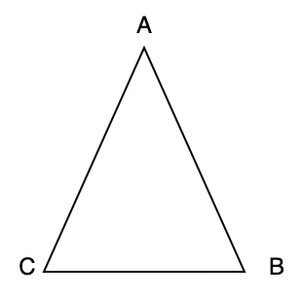In the above figure, ABC is a triangle. AB, BC, and AC are three sides of a triangle, ∠A, ∠B and ∠C are the angles of this triangle. Let’s study some properties and inequalities of triangles.

### Isosceles Triangle

A triangle in which two sides are equal is called an isosceles triangle. So, ∆ABC has AB = AC, the word ‘Isosceles’ means “Two legs”, therefore, in a Triangle too, when two legs/two sides are equal, the triangle is known as Isosceles Triangle. Out of the well-known Isosceles triangles, one is ‘Right Isosceles triangle’ where one angle is 90° and the two sides (base and perpendicular) are equal.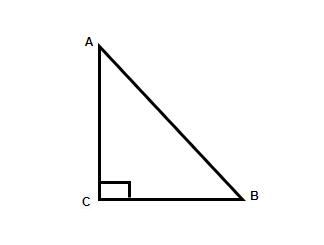Example of Isosceles Triangle: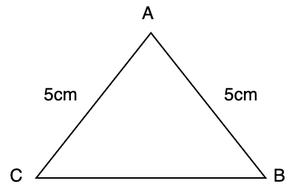### Theorem:Angles opposite to equal sides in an isosceles triangle are equal.

Proof: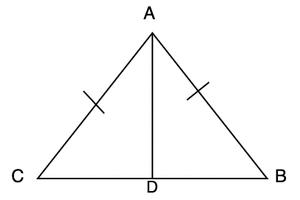We need to prove that ∠B and ∠C are equal. Let’s draw bisector of ∠A and join it to BC.

AB = AC,

So, the two triangles are congruent.

So, ∠B and ∠C are corresponding angles of congruent triangles. Thus, they are equal.

Is the converse of this theorem also true? If two angles of a triangle are equal, that means the sides opposite to them are also equal?

Yes, the converse is also true, this theorem can be proved in a similar manner, by using the ASA congruence rule.

Let’s solve some proof-related problems regarding these properties:

Question 1: E and F are respectively the mid-points of equal sides AB and AC of Δ ABC (see figure). Show that BF = CE.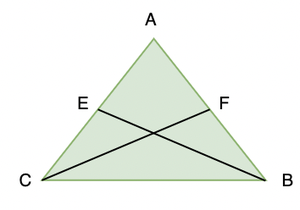Solution:

In Δ ABF and Δ ACE,

AB = AC (Given)

∠ A = ∠ A (Common)

AF = AE (Halves of equal sides)

So, Δ ABF ≅ Δ ACE (SAS rule)

Therefore, BF = CE (CPCT)

Question 2: In an isosceles triangle ABC with AB = AC, D, and E are points on BC such that BE = CD. Show that AD = AE.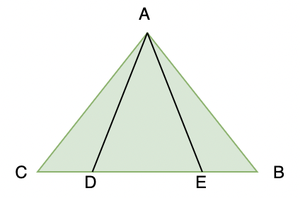Solution:

In Δ ABD and Δ ACE,

AB = AC (Given)

∠ B = ∠ C (Angles opposite to equal sides)

Also, BE = CD

So, BE – DE = CD – DE

That is, BD = CE

So, Δ ABD ≅ Δ ACE(Using SAS Rule)

Hence, Proved.

### Equilateral Triangles

The triangles in which all three sides are equal are called equilateral triangles. All three angles of an Equilateral Triangle are equal and since the sum of all the angles is 180°, each angle is 60° respectively. It is important to note that all Equilateral triangles are always similar to each other.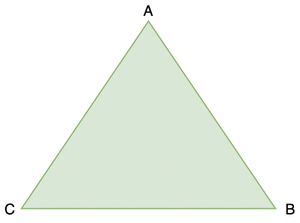### Theorem: If ABC is an equilateral triangle and P is a point on the arc BC of the circumcircle of the triangle ABC, then; PA = PB + PC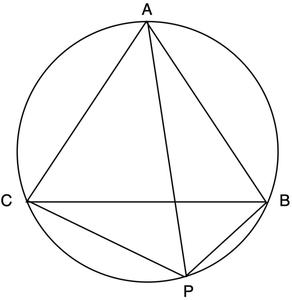Proof:

For a cyclic quadrilateral ABPC, we have;

PA.BC=PB.AC+PC.AB

For an equilateral triangle ABC,

AB = BC = AC

So, PA.AB = PB.AB+PC.AB

Taking AB as a common;

PA.AB = AB(PB+PC)

PA = PB + PC

Hence, proved.

### Ordering Triangle Sides and Angles

We can order sides in increasing order of length if angles are given to us. Similarly, angles of the triangle can be ordered. Properties that largest side is directly opposite to largest angle and so on come to our rescue.

Question: In the triangle, ABC given below, order the sides from shortest to longest using the given angles.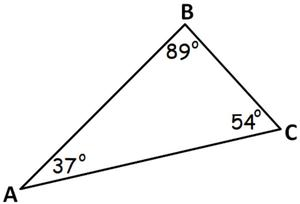In any triangle, the smallest angle is always opposite to the shortest side and the largest angle is always opposite to the longest side and vice versa. In the triangle ABC given above, because angle A is the smallest angle, the side BC must be the shortest side. And also, because angle B is the largest angle, the side AC must be the longest side. Let’s put it all together. Hence, the order of the three sides from shortest to longest is side BC, side AB, and side AC

### Triangle Inequality Theorem

So far, we have been focused on the equality of sides and angles of a triangle or triangles. Sometimes, we do come across unequal objects, we need to compare them.

Theorem 1: If two sides of a triangle are unequal, then the angle opposite to the larger side is larger.

Theorem 2: In any triangle, the side opposite to the larger (greater) angle is longer. It is the converse of Theorem 1 stated above.

Theorem 3: Sum of any two sides of a triangle is always greater than the third side.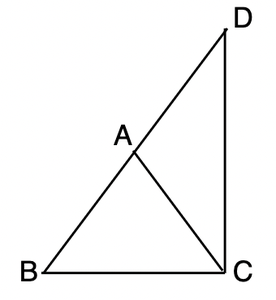Proof:

Notice that the side BA of Δ ABC has been produced to a point D such that AD = AC. Now, since ∠BCD > ∠BDC.

By the properties mentioned above, we can conclude that BD > BC.

We know that, BD = BA + AD

So, BA + AD > BC

= BA + AC > BC

So, this proved sum of two sides triangle is always greater than the other side.

Question: D is a point on side BC of triangle ABC such that AD = DC. Show that AB > BD.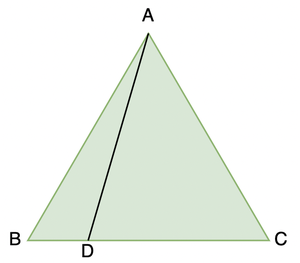Solution:

In triangle DAC,

∠ADC = ∠ACD (Angles opposite to equal sides)

∠ ADC is an exterior angle for ΔABD.